# Monthly Challenge – Sofia Air – Solution – Banana

1

Air pollution is one of the major global topics in every part of the modern life – politics, ecology, social responsibility etc. In the last few years it became a major topic for Bulgaria and especially for Sofia. It is a well – known fact that our capital’s air is rather polluted. The reasons for that pollution vary from social to environmental. The air pollution is measured in particulate matter (PM) which indicates the mixture of solid particles and liquid droplets found in the air. One thing is certain – measures should be taken.

Considering the fact that Sofia is a fast-growing agglomeration, it is clear that the pollution will also grow. Maybe even faster that the citizens and urbanization process.

Information is the strongest priority of our time and it really important to use it in the best possible way. The main aim of this study is to properly investigate the information which is available for Sofia’s from different kind of sources which measure the air pollution. Our target is to create a model predicting the air pollution in the next 24 hours for Sofia.

2. Data understanding

The data which is provided for the study is:
• Official air quality measurements (5 stations in the city) – the data is gathered based on the EU guidelines which is very important in our case considering the fact that Bulgaria is part of the European Union. It is important to keep in mind that there are only 5 stations in the city which cover particular parts of the city.
• Citizen science air quality measurements – unofficial data marked with geohash which requires careful cleaning and consideration
• Meteorological measurements (1 station) – it covers the period between 2012 and 2018
• Topography data

3.Data Preparation

After taking a detail look at all the datasets, our team decided to change the method of investigation from the start. Our first step in preparing the data was set to be processing the EEA data. We join the official data together with the Topography data and we check the missing values in the data set which is very important in every approach.

There are two ways for looking into the data – daily and hourly. To make a decision we have to explore the two options and we plot the results.

`NAsbyCol <- as.data.frame(sapply(EEAData, function(x) length(which(is.na(x)))))`

```#Get the meta data EEAData <- left_join(EEAData,EEADataMeta, by = c("AirQualityStation" = "AirQualityStation"))```

```#Check the time intervals checkTimeIntervals <- EEAData %>% group_by(AveragingTime) %>% summarise(count = n()) #check the records daily vs hourly```

```#only daily measures EEADataDaily <- EEAData[EEAData\$AveragingTime == "day",] head(EEADataDaily\$DatetimeEnd, 10) EEADataDaily\$DatetimeEnd <- ymd_hms(EEADataDaily\$DatetimeEnd,tz="Europe/Athens") EEADataDaily\$DatetimeBegin <- ymd_hms(EEADataDaily\$DatetimeBegin,tz="Europe/Athens") head(EEADataDaily\$DatetimeEnd, 10) sapply(EEADataDaily,class)```

```EEADataDaily <- distinct(EEADataDaily[,c("AirQualityStation","DatetimeBegin","DatetimeEnd","Concentration","CommonName","Latitude","Longitude")]) %>% arrange(AirQualityStation,DatetimeEnd) windows() ggplot(data = EEADataDaily, aes( x = DatetimeEnd, y = Concentration, group = AirQualityStation, colour = AirQualityStation)) + geom_line() + facet_wrap("AirQualityStation")```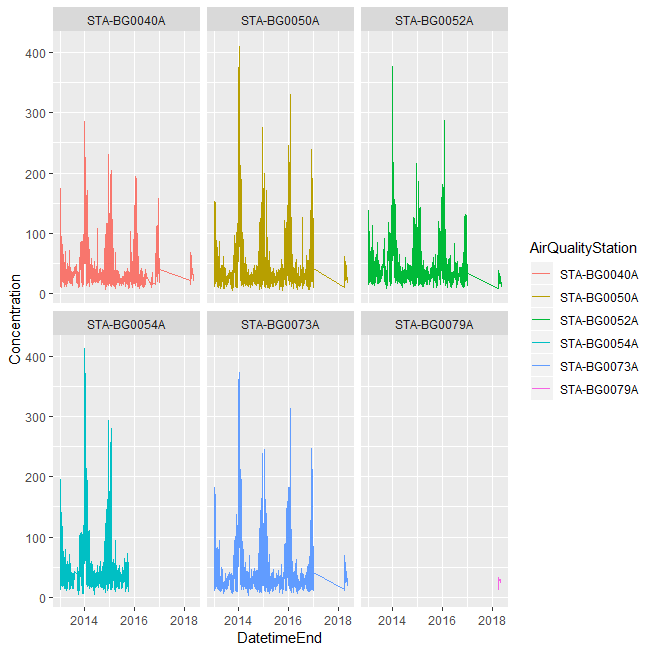Here we can see that 4 of the station has missing values in the period 2016 – 2018. One of the station have no values at all before 2018 and one of them has stopped measuring after the mid of 2016. It is important to clarify that these gaps in the measurements are because the stations probably didn’t record anything on a daily basis. That is the main reason to look into the data through hour measurements.
We are looking to the data – hourly based. We made a check for duplicates and result was none. The results are once again plotted.

```#keep only hourly measures EEAData <- EEAData[EEAData\$AveragingTime == "hour",] NAsbyCol <- as.data.frame(sapply(EEAData, function(x) length(which(is.na(x))))) head(EEAData\$DatetimeEnd,10) EEAData\$DatetimeEnd <- ymd_hms(EEAData\$DatetimeEnd,tz="Europe/Athens") EEAData\$DatetimeBegin <- ymd_hms(EEAData\$DatetimeEnd,tz="Europe/Athens") head(EEAData\$DatetimeEnd,10) sapply(EEAData,class) NAsbyCol <- as.data.frame(sapply(EEAData, function(x) length(which(is.na(x))))) EEAData <- distinct(EEAData[,c("AirQualityStation","DatetimeEnd","Concentration")]) %>% arrange(AirQualityStation,DatetimeEnd)```

```#Check for doubles checkDoubles <- EEAData %>% group_by(AirQualityStation,DatetimeEnd) %>% summarise(count = n()) %>% dplyr::filter(count > 1) #there are no duplicates```

```stationNames <- unique(EEAData\$AirQualityStation) windows() ggplot(data = EEAData, aes( x = DatetimeEnd, y = Concentration, group = AirQualityStation, colour = AirQualityStation)) + geom_line() + facet_wrap("AirQualityStation")```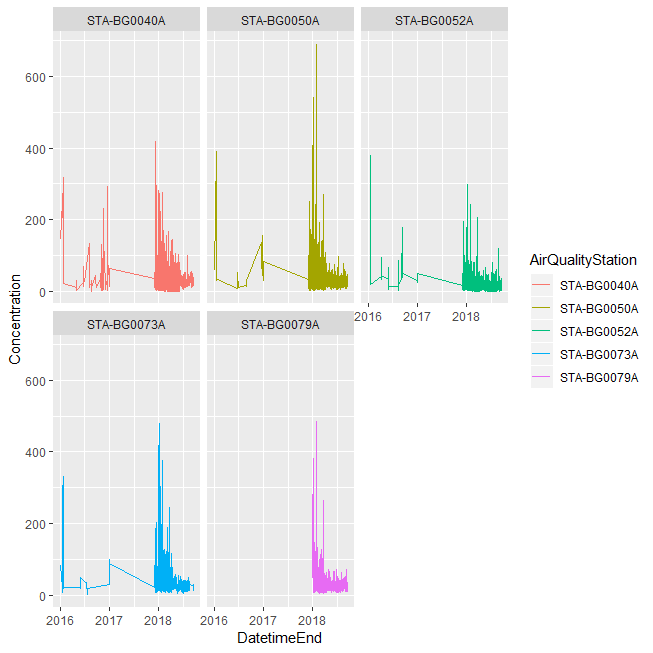Looking at the plots it appears that till the beginning of 2018, the official European stations had measured the P10 pollution for a day and in 2018 the records are per hour.
Since we see that we have full hourly data only In the last we period, we take it separately and remove the hourly measurements before June 2017.

```stationNames <- unique(EEAData\$AirQualityStation) windows() ggplot(data = EEAData, aes(x = DatetimeEnd, y = Concentration, group = AirQualityStation, colour = AirQualityStation)) + geom_line() + facet_wrap("AirQualityStation")```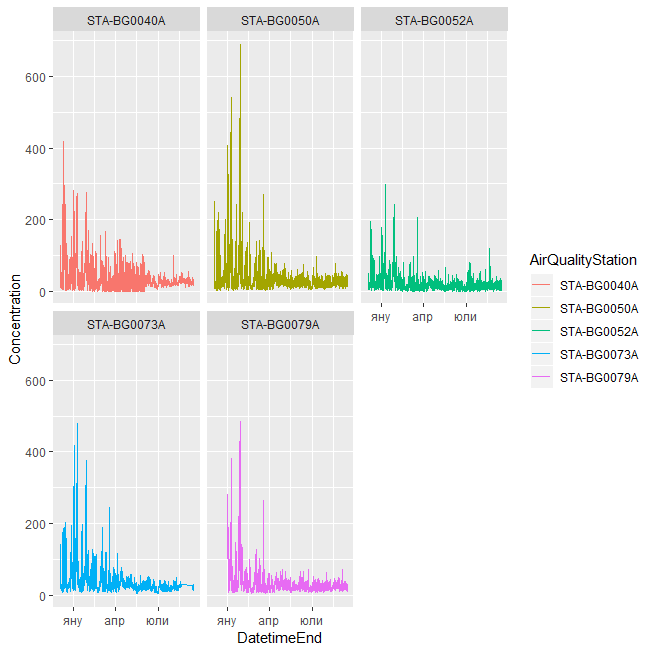Our main purpose is to have clear idea of the measurements from the stations in order to interpolate in the most efficient way. The final step of processing the EEA data is to map the location of the stations in Sofia which will be very important in the further investigation and clustering of the citizen data.
#Split EEA data into stations

```EEADataList <- vector("list", length(stationNames)) names(EEADataList) <- stationNames```

```for (i in stationNames){ EEADataList[[i]] <- EEAData[EEAData\$AirQualityStation == i,] }```

```# Genrate a list of time vectors on hourly sampling rate hourVectorList <- vector("list", length(stationNames)) names(hourVectorList) <- stationNames for (i in stationNames){ hourVectorList[[i]]=as.data.frame(seq.POSIXt(from=min(EEADataList[[i]]\$DatetimeEnd),to=max(EEADataList[[i]]\$DatetimeEnd), by="hour")) colnames(hourVectorList[[i]])="DatetimeEnd" hourVectorList[[i]]=left_join(hourVectorList[[i]],EEADataList[[i]],by="DatetimeEnd") if(sum(duplicated(hourVectorList[[i]]\$DatetimeEnd)) > 0){ print("Duplicates") } } # NO duplicates```

```EEADataInterpolationBasics <- vector("list", length(stationNames)) names(EEADataInterpolationBasics) <- stationNames```

```#create a vector for the umpputing imputedEEADataList <- vector("list", length(stationNames)) names(imputedEEADataList) <- stationNames```

```for (i in stationNames){ EEADataInterpolationBasics[[i]] <- vector("list", 3) variablesOfInterest <- c("statsNA", "plotNA.distribution", "plotNA.imputations") names(EEADataInterpolationBasics[[i]]) <- variablesOfInterest EEADataInterpolationBasics[[i]][] <- statsNA(hourVectorList[[i]]\$Concentration) EEADataInterpolationBasics[[i]][] <- plotNA.distribution(hourVectorList[[i]]\$Concentration, main = i) imputedEEADataList[[i]] <- na.kalman(hourVectorList[[i]]\$Concentration) EEADataInterpolationBasics[[i]][] <- plotNA.imputations(hourVectorList[[i]][,"Concentration"], imputedEEADataList[[i]],main = i) hourVectorList[[i]]\$imputedConcentration <- imputedEEADataList[[i]] hourVectorList[[i]]\$AirQualityStation <- i } #rm(imputedEEADataList)```

```window() plotNA.imputations(hourVectorList[][,"Concentration"], imputedEEADataList[],main = 1)```

```window() plotNA.distribution(hourVectorList[]\$Concentration, main = 1) #dev.off(dev.list()["RStudioGD"])```

```#Plot Official stations windows() ggmap(WatercolorMap) + geom_point(data = EEADataMeta, aes(x = Longitude, y = Latitude), color = "blue",size = 3) + geom_text(data = EEADataMeta, aes(x = Longitude, y = Latitude, label=CommonName),hjust=0, vjust=0)```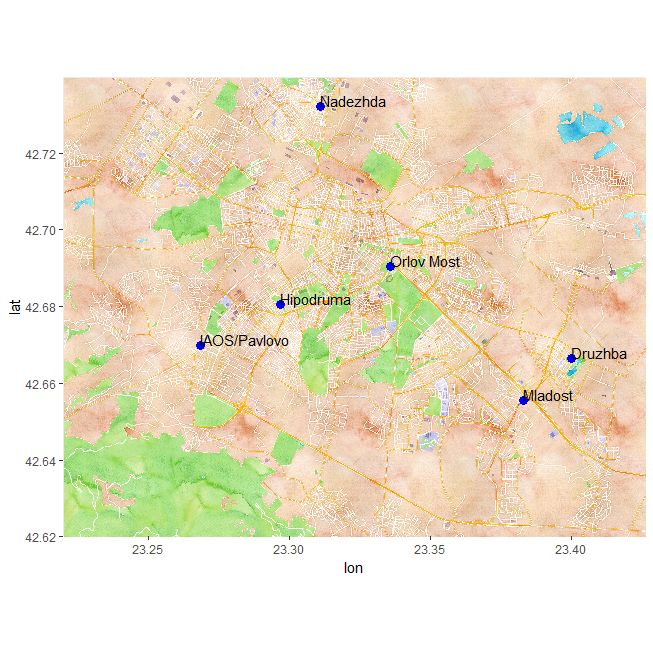We furtherly impute the Meteo date via the Kalman Method.
The next step is to take a careful look at the citizen data. Firstly, we transform the date (which is in character format) in a date-time format and check the classes of the variables. When we are ensured that all the variables are with the correct classes we merge the two datasets for 2017 and 2018 and define the unique stations. We further check for missing values in the unique ones.

```#Transofrm time in Data2017 and Data2018 into POSIXct and check the classes again Data2017 <- mutate(Data2017,time = ymd_hms(time)) Data2018 <- mutate(Data2018,time = ymd_hms(time)) sapply(Data2017,class) sapply(Data2018,class) #Merge the two datasets DataAll <- bind_rows(Data2017,Data2018) sapply(DataAll,class) #Unique stations on the combined dataset uniqueStations <- unique(DataAll\$geohash) NAsbyCol <- as.data.frame(sapply(DataAll, function(x) length(which(is.na(x))))) DataAll <- DataAll[is.na(DataAll\$geohash) == 0,]```

With this part we check which of the station has no GEOhashes and remove them from the set. We decode the GEOhashes of each unique station and use coordinates do define which stations are in the observed area and in which point exactly. In that way we remove all the station out of Sofia and keep only the ones we are actually going to use for the model.
#Decode the geohashes

```decodedHashes <- gh_decode(DataAll\$geohash) #Merge with the data DataAll <- bind_cols(DataAll,decodedHashes[,c("lat","lng")]) #Using the function point.is.polygon we create a vector which shows if the coordiantes are in the poligon defined by the Sofia Topography map #Get coordinates of the stations xOfStations = as.vector(decodedHashes\$lng) yOfStations = as.vector(decodedHashes\$lat) #Get coordinates of Sofia xOfSofia <- as.vector(SofiaTopography\$Lon) yOfSofia <- as.vector(SofiaTopography\$Lat) #Create the vector isStationInSofia <- as.vector(point.in.polygon(xOfStations, yOfStations, xOfSofia, yOfSofia)) DataAllSofia <- DataAll[which(isStationInSofia == 1),]```

Since the dataset contains a lot of measurements, important thing to do is to look for duplicates which in the particular case means if there are more than one record for a station in one moment. The answer was yes so as a solution we took the average from the duplicates measurements.

```#Look at and take care of duplicates Duplicates <- DataAllSofia %>% group_by(geohash,time) %>% summarise(countObs = n()) %>% dplyr::filter(countObs > 1)```

```LookAtDuplicates <- left_join(Duplicates,DataAllSofia,by = c("geohash", "time")) %>% arrange(geohash,time)```

```DataAllSofia <- DataAllSofia %>% group_by(geohash,time) %>% summarise(P1 = mean(P1), P2 = mean(P2), temperature = mean(temperature), humidity = mean(humidity), pressure = mean(pressure), lat = mean(lat), lng = mean(lng))```

Our next approach is to handle the mismeasurements in the data. For this purpose we create two test data.frames in which we select the stations that have records for temperature, humidity and pressure all equals 0 which is rather impossible. We removed these records and retain only 9 ones where the pressure is more than 0.

```#We are starting to substitute the mismeasured valeus with NA test1 <- DataAllSofia[DataAllSofia\$temperature == 0 & DataAllSofia\$humidity == 0,] test2 <- DataAllSofia[DataAllSofia\$temperature == 0 & DataAllSofia\$humidity == 0 & DataAllSofia\$pressure > 0,] rm(test1,test2) #NA for the 0 zeroes from the tests DataAllSofia <- DataAllSofia %>% mutate(pressure = ifelse(pressure == 0, NA, pressure), humidity = ifelse(humidity == 0 & temperature == 0, NA, humidity), temperature = ifelse(humidity == 0 & temperature == 0, NA, temperature)) NAsbyCol <- as.data.frame(sapply(DataAllSofia, function(x) length(which(is.na(x)))))```

We decided to look at the basic stats of the humidity in the set since we had the feeling since our first approach that it won’t be a good predictable variable. The basic statistics for the humidity measurements show that the records are very similar and the variable has no added value since the median and the mean are almost the same number and the standard deviation is very small compared to the mean.

X..MeteoData.PSLAVG
nobs 2.452000e+03
NAs 0.000000e+00
Minimum 9.888255e+02
Maximum 1.039452e+03
1. Quartile 1.012530e+03
3. Quartile 1.020658e+03
Mean 1.016735e+03
Median 1.016086e+03
Sum 2.493035e+06
SE Mean 1.363960e-01
LCL Mean 1.016468e+03
UCL Mean 1.017003e+03
Variance 4.561696e+01
Stdev 6.754033e+00
Skewness 2.147240e-01
Kurtosis 6.299400e-01

For further cleaning of the data we clean all the obvious outliers in the variables. We set the thresholds by adding ¼ to the minimum and to the maximum measurement of each variable in the EEA data. In this way we clean the records which cannot be adequate for variable reasons and we use the official stations as a point for comparison for clear measurements.

```DataAllSofia <- DataAllSofia %>% mutate(pressure = ifelse(PSLMAX * 100 + (PSLMAX - PSLMIN) * 0.25 * 100 < pressure | PSLMIN * 100 - (PSLMAX - PSLMIN) * 0.25 * 100 > pressure,NA,pressure), humidity = ifelse(RHMAX + (RHMAX - RHMIN) * 0.25 < humidity | RHMIN - (RHMAX - RHMIN) * 0.25 > humidity,NA,humidity), temperature = ifelse(TASMAX + (TASMAX - TASMIN) * 0.25 < temperature | TASMIN - (TASMAX - TASMIN) * 0.25 > temperature,NA,temperature))```

Since our predictive variable is P10, we took a deeper look at its measurements. It appeared that there are some station which has only 0 values for P10 and we removed them from the set together with the four station with rather high percentage.

```CheckP1Zeroes <- DataAllSofia %>% group_by(geohash) %>% summarise(obs = n(), P1Zeroes = sum(ifelse(P1 == 0,1,0)), PercP1Zeroes = P1Zeroes / obs) %>% arrange(PercP1Zeroes) windows() plot(CheckP1Zeroes\$PercP1Zeroes)```

`#we cut out everything above 100 % and the 4 outliers`

```notProperMeasurments <- CheckP1Zeroes[CheckP1Zeroes\$PercP1Zeroes > 0.2,"geohash"] DataAllSofia <- DataAllSofia[!(DataAllSofia\$geohash %in% notProperMeasurments\$geohash),]```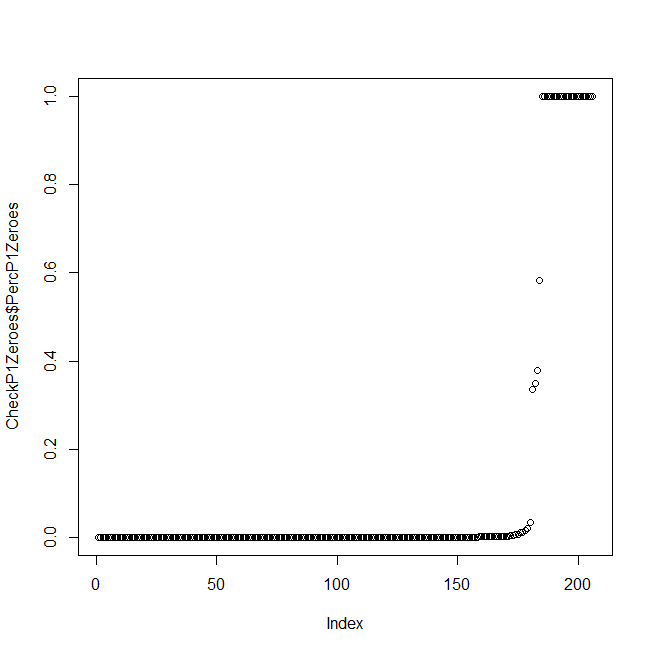We reached the point where we merged the official dataset with the citizen one. Since we are well aware that there a lot of mismeasurements of P10 in the citizen data, once again will use the EEA stations as a benchmark for the records. We set difference between the maximum P10 for a day in the official stations and in the citizen ones. Out main idea is not to cut out these results but to use them as a sign that the air was polluted no matter with how much. The thresholds are with 100 and 50 above the measured in EEA data for a day.

```#check where P1 is above the max in EEA data DataAllSofia1 <- DataAllSofia %>% mutate(P1 = ifelse(P1 >= maxConcentration,NA,P1)) NAsbyCol <- as.data.frame(sapply(DataAllSofia1, function(x) length(which(is.na(x))))) ```

```#74534 if P1 > max DataAllSofia1 <- DataAllSofia %>% mutate(P1 = ifelse(P1 >= maxConcentration + 50,NA,P1)) NAsbyCol <- as.data.frame(sapply(DataAllSofia1, function(x) length(which(is.na(x))))) ```

```#16055 if P1 > max + 50 DataAllSofia1 <- DataAllSofia %>% mutate(P1 = ifelse(P1 >= maxConcentration + 100,NA,P1)) NAsbyCol <- as.data.frame(sapply(DataAllSofia1, function(x) length(which(is.na(x)))))```

In the next two graphics we visualize the stations which had measured 50 or 100 p10 above the official for the day. The goal is to see where exactly they are located and to find logic which of them had probably measured true by pattern of their location. As a result we decided to cap all the measures at maximum daily p10 plus 100.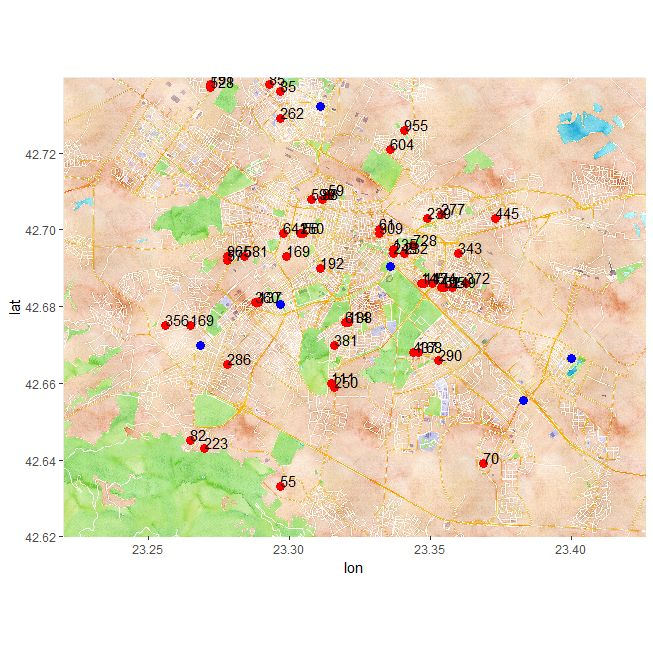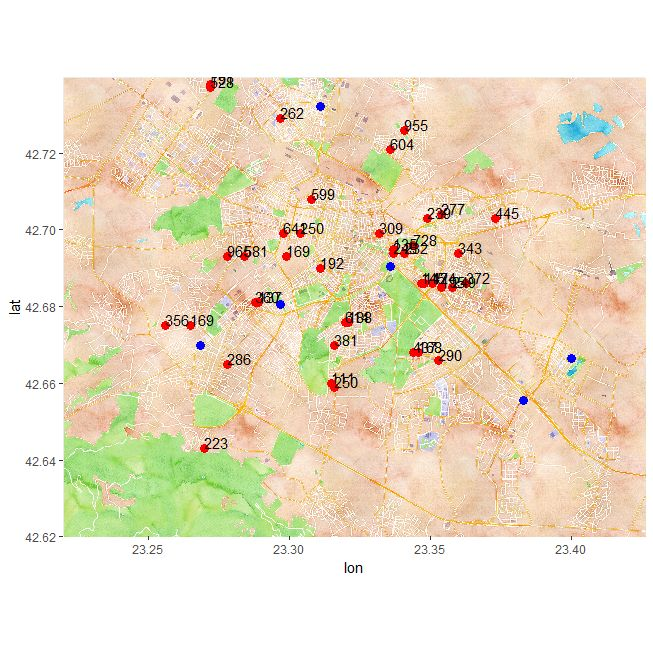The next step of cleaning is to remove all the station from citizen data with less than 90 days full observations and we plot (with the red dots) to see which stations had dropped down. We consider it a good decision since all the station we cut are close enough to several others so we won’t lose any valuable information.

```#Remove obs with less than 90 days GroupedDataSofiaGeohash <- DataAllSofia %>% group_by(geohash) %>% summarise(obs = n(), tmin = min(time), tmax = max(time), days = as.numeric(tmax - tmin), lat = mean(lat), lng = mean(lng)) %>% arrange(days,geohash)```

`removeNotSerious <- GroupedDataSofiaGeohash[GroupedDataSofiaGeohash\$obs <= 90 | GroupedDataSofiaGeohash\$days <= 90,c("geohash","lng","lat")]`

```windows() ggmap(WatercolorMap) + geom_point(data = removeNotSerious, aes(x = lng, y = lat), color = "red",size = 2) + geom_point(data = GroupedDataSofiaGeohash[!(GroupedDataSofiaGeohash\$geohash %in% removeNotSerious\$geohash),], aes(x = lng, y = lat), color = "blue")```

After this we proceed with the creation of clusters for the cirtizen data.

First we will find the closed topographical point and assign the elevation from there
``` GroupedDataSofiaGeohash <- DataAllSofia %>% group_by(geohash) %>% summarise(obs = n(), tmin = min(time), tmax = max(time), days = as.numeric(tmax - tmin), lat = mean(lat), lng = mean(lng)) %>% arrange(days,geohash) GroupedDataSofiaGeohash\$nearest_topo <- apply(gDistance(SpatialPoints(SofiaTopography[,c("Lon","Lat")]), SpatialPoints(GroupedDataSofiaGeohash[,c("lng","lat")]), byid=TRUE), 1, which.min) GroupedDataSofiaGeohash\$Elevation <- SofiaTopography[GroupedDataSofiaGeohash\$nearest_topo,"Elev"] DataAllSofia <- left_join(DataAllSofia,GroupedDataSofiaGeohash[,c("geohash","Elevation")], by = "geohash") ```
Note that using the nearest_topo column we can assign the citizen data to clusters as was outlined in the mentor’s notes, however we believe that this approach is not the correct one.

Before we continue with potential grouping we want to create an automated method to assess the quality of the citizen data.
We have decided to use the following:
First we create a “list of closeness”, which finds all the stations within a certain distance from a chosen station. Then in the so created groups we calculate the difference between the P1 measurments for all the hourly measurments, which the stations have in common.
In the code below, we have chosen a distance of 0.01(as calculated by gDistance), calcualted the difference as the sum of the absolute differences of P1 measurments divided by the number of common observations and requesting that the common observations are at least 200. The 200 and 0.01 were chosen arbitrary and experimenting with them and other improvements of the method should be cosidered, however we were more focused on a “proof of concept”.
``` citizenGeohash <- GroupedDataSofiaGeohash\$geohash DistanceBetweenCitizenStations <- as.data.frame(gDistance(SpatialPoints(GroupedDataSofiaGeohash[,c("lng","lat")]), byid=TRUE)) ListOfCloseness <- apply(DistanceBetweenCitizenStations,1,function (x) which(x < 0.01)) names(ListOfCloseness) <- citizenGeohash```
``` AbsDiffBetweenCitizens <- vector("list", length(citizenGeohash)) names(AbsDiffBetweenCitizens) <- citizenGeohash AbsDiffBetweenStationsCount <- vector("list", length(citizenGeohash)) names(AbsDiffBetweenStationsCount) <- citizenGeohash```
``` InnerJoins <- vector("list", length(citizenGeohash)) names(InnerJoins) <- citizenGeohash```
``` AbsDiffBetweenCitizensDataframe <- data.frame(matrix(NA,length(citizenGeohash),length(citizenGeohash))) names(AbsDiffBetweenCitizensDataframe) <- seq(1:length(citizenGeohash)) row.names(AbsDiffBetweenCitizensDataframe) <- seq(1:length(citizenGeohash)) AbsDiffBetweenCitizensCountDataframe <- data.frame(matrix(NA,length(citizenGeohash),length(citizenGeohash))) names(AbsDiffBetweenCitizensCountDataframe) <- seq(1:length(citizenGeohash)) row.names(AbsDiffBetweenCitizensCountDataframe) <- seq(1:length(citizenGeohash)) ```
``` for (i in 1:length(citizenGeohash)){ InnerJoins[[i]] <- vector("list", length(ListOfCloseness[[i]])) z = 0 AbsDiffBetweenCitizens[[i]] <- data.frame(matrix(NA,1,1)) names(AbsDiffBetweenCitizens[[i]]) <- "empty" row.names(AbsDiffBetweenCitizens[[i]]) <- i AbsDiffBetweenStationsCount[[i]] <- data.frame(matrix(NA,1,1)) names(AbsDiffBetweenStationsCount[[i]]) <- "empty" row.names(AbsDiffBetweenStationsCount[[i]]) <- i geo1 <- GroupedDataSofiaGeohash[i,"geohash"] for (j in c(ListOfCloseness[[i]])){ z = z + 1 geo2 <- GroupedDataSofiaGeohash[j,"geohash"] a <- DataAllSofia[DataAllSofia\$geohash == geo1\$geohash,c("time","P1")] b <- DataAllSofia[DataAllSofia\$geohash == geo2\$geohash,c("time","P1")] comb <- inner_join(a,b, by = c("time")) InnerJoins[[i]][[z]] <- comb if (dim(comb) == 0){ combDiff <- as.data.frame(NA) names(combDiff) <- j bleh <- as.data.frame(NA) names(bleh) <- j AbsDiffBetweenCitizens[[i]] <- bind_cols(AbsDiffBetweenCitizens[[i]],combDiff) AbsDiffBetweenStationsCount[[i]] <- bind_cols(AbsDiffBetweenStationsCount[[i]],bleh) } else{ AbsDiffBetweenCitizensDataframe[i,j] <- ifelse(nrow(comb) < 200,NA,sum(abs(comb[,2] - comb[,3]),na.rm = TRUE) / nrow(comb)) AbsDiffBetweenCitizensCountDataframe[i,j] <- nrow(comb) combDiff <- as.data.frame(ifelse(nrow(comb) < 200,NA,sum(abs(comb[,2] - comb[,3]),na.rm = TRUE) / nrow(comb))) names(combDiff) <- j bleh <- as.data.frame(nrow(comb)) names(bleh) <- j AbsDiffBetweenCitizens[[i]] <- bind_cols(AbsDiffBetweenCitizens[[i]],combDiff) AbsDiffBetweenStationsCount[[i]] <- bind_cols(AbsDiffBetweenStationsCount[[i]],bleh) } } } ```

The end result are two matrixes, one containg the calcualted differences and the other the number of common observations.
Once we have this we continue with the pruning. The pruning is done the following way:
First we find the stations with the highest number of differences bigger than 10 with its assigned cluster.
``` ListOfDifferencesNew <- apply(AbsDiffBetweenCitizensDataframe,1,function (x) length(which(x > 10))) x <- max(ListOfDifferencesNew) y <- which(ListOfDifferencesNew == x) ```
Again the number 10 was chosen arbitrary. After this we remove those stations and repeat the process until there are no stations which have more than 1(chosen arbitraly) differences above 10 with their respective clusters.
``` citizenGeohashNew <- citizenGeohash DistanceBetweenCitizenStationsNew <- DistanceBetweenCitizenStations runs = 0 while(x > 1){ citizenGeohashNew <- citizenGeohashNew[-y] DistanceBetweenCitizenStationsNew <- DistanceBetweenCitizenStationsNew[-y,-y] ListOfClosenessNew <- apply(DistanceBetweenCitizenStationsNew,1,function (x) which(x < 0.01)) names(ListOfClosenessNew) <- citizenGeohashNew```
``` AbsDiffBetweenCitizensNew <- vector("list", length(citizenGeohashNew)) names(AbsDiffBetweenCitizensNew) <- citizenGeohashNew AbsDiffBetweenStationsCountNew <- vector("list", length(citizenGeohashNew)) names(AbsDiffBetweenStationsCountNew) <- citizenGeohashNew```

InnerJoinsNew <- vector(“list”, length(citizenGeohashNew))
names(InnerJoinsNew) <- citizenGeohashNew

``` AbsDiffBetweenCitizensDataframeNew <- data.frame(matrix(NA,length(citizenGeohashNew),length(citizenGeohashNew))) names(AbsDiffBetweenCitizensDataframeNew) <- seq(1:length(citizenGeohashNew)) row.names(AbsDiffBetweenCitizensDataframeNew) <- seq(1:length(citizenGeohashNew)) AbsDiffBetweenCitizensCountDataframeNew <- data.frame(matrix(NA,length(citizenGeohashNew),length(citizenGeohashNew))) names(AbsDiffBetweenCitizensCountDataframeNew) <- seq(1:length(citizenGeohashNew)) row.names(AbsDiffBetweenCitizensCountDataframeNew) <- seq(1:length(citizenGeohashNew))```
``` for (i in 1:length(citizenGeohashNew)){ InnerJoinsNew[[i]] <- vector("list", length(ListOfClosenessNew[[i]])) z = 0 AbsDiffBetweenCitizensNew[[i]] <- data.frame(matrix(NA,1,1)) names(AbsDiffBetweenCitizensNew[[i]]) <- "empty" row.names(AbsDiffBetweenCitizensNew[[i]]) <- i AbsDiffBetweenStationsCountNew[[i]] <- data.frame(matrix(NA,1,1)) names(AbsDiffBetweenStationsCountNew[[i]]) <- "empty" row.names(AbsDiffBetweenStationsCountNew[[i]]) <- i geo1 <- GroupedDataSofiaGeohash[i,"geohash"] for (j in c(ListOfClosenessNew[[i]])){ z = z + 1 geo2 <- GroupedDataSofiaGeohash[j,"geohash"] a <- DataAllSofia[DataAllSofia\$geohash == geo1\$geohash,c("time","P1")] b <- DataAllSofia[DataAllSofia\$geohash == geo2\$geohash,c("time","P1")] comb <- inner_join(a,b, by = c("time")) InnerJoinsNew[[i]][[z]] <- comb if (dim(comb) == 0){ combDiff <- as.data.frame(NA) names(combDiff) <- j bleh <- as.data.frame(NA) names(bleh) <- j AbsDiffBetweenCitizensNew[[i]] <- bind_cols(AbsDiffBetweenCitizensNew[[i]],combDiff) AbsDiffBetweenStationsCountNew[[i]] <- bind_cols(AbsDiffBetweenStationsCountNew[[i]],bleh) } else{ AbsDiffBetweenCitizensDataframeNew[i,j] <- ifelse(nrow(comb) < 200,NA,sum(abs(comb[,2] - comb[,3]),na.rm = TRUE) / nrow(comb)) AbsDiffBetweenCitizensCountDataframeNew[i,j] <- nrow(comb) combDiff <- as.data.frame(ifelse(nrow(comb) < 200,NA,sum(abs(comb[,2] - comb[,3]),na.rm = TRUE) / nrow(comb))) names(combDiff) <- j bleh <- as.data.frame(nrow(comb)) names(bleh) <- j AbsDiffBetweenCitizensNew[[i]] <- bind_cols(AbsDiffBetweenCitizensNew[[i]],combDiff) AbsDiffBetweenStationsCountNew[[i]] <- bind_cols(AbsDiffBetweenStationsCountNew[[i]],bleh) } } } ListOfDifferencesNew <- apply(AbsDiffBetweenCitizensDataframeNew,1,function (x) length(which(x > 10))) x <- max(ListOfDifferencesNew) y <- which(ListOfDifferencesNew == x) runs = runs + 1 } ```
After this we are left with 100 stations from a starting point of 148.
The next step should be creating clusters with those pruned stations. Unfortunately we couldn’t come up with an algorithm, which we find satisfying enough even as a proof of concept, therefore we will stop at this point.

#### 11 thoughts on “Monthly Challenge – Sofia Air – Solution – Banana”

1.junior says:
0

Please, import your code as selectable text, we have great capabilities here on the platform to render jupyter notebook, or at least past it as text in quted field or something…. using images to show code is very lame

2.junior says:
0

Your assignments to peer review (and give feedback below the coresponding articles) for week 1 of the Monthly challenge are the following teams:
https://www.datasciencesociety.net/sofia-air-week-1/
https://www.datasciencesociety.net/monthly-challenge-sofia-air-solution-jacob-avila/
https://www.datasciencesociety.net/monthly-challenge-sofia-air-solution-newbees/

3.sonyailieva says:
0

Pumpkin team: We would like first to congratulate you, Banana team, for your first great written article! The first two parts about the business and data understanding are great introduction of the goal of the Monthly challenge and about the main aspects of the issue which we are dealing with – the air pollution in the capital of Bulgaria. We appreciate the simple and clear operations that you have used in your code because it is easy for the others to follow your steps and logic as well as to help those who do not fully understand the functions used in the code. It is obvious that you have given much effort and managed to apply a big variety of R commands. We do not have any remarks for improvements at this stage but only would like to ask about one of the filters you intend to apply – why do you want to filter to the stations which are missing in 2018 and why you checked whether there are some missing both in 2017 and 2018? Our approach, for example, is to look at the latest data (excluding the stations which do not appear in 2018) because in order to make a forecast we want to use the latest information. Maybe you meant the same but we just want to double-check. 🙂

4.kams says:
0

Great work! From the writing, I infer you are a local team in Sofia. I appreciate your passion for the problem. I loved the fact that you chose to keep all the data. this is so important, IMHO, to not delete data too early.

I am not familiar with R. However, explanation assumed the reader knows R. So your points about time data seemed specific to your tool set.

Good work over all.

5.junior says:
0

Your assignments to peer review (and give feedback below the coresponding articles) for week 2 of the Monthly challenge are the following teams:
https://www.datasciencesociety.net/sofia-air-quality-eda-exploratory-data-analysis/
https://www.datasciencesociety.net/air-quality-week-1/
https://www.datasciencesociety.net/monthly-challenge-sofia-air-solution-sky_data/

6.beartooth says:
0

Good that you looked at the consistency of the pressure, temp and humidity, but what about the air pollution measurements?
I don’t think that excluding of observations is a good idea, as this means that the time series will not be continuous. Did you consider replacing the strange values with the mean or some other assumed value?
How did you decide on the number of clusters? Why 10?

7.iliyanski says:
0

Hi team,
You have done excellent job this weekl! No doubt that your work is great. I think all of us, or at least I am going to learn from you. What I like about your article is that it is very easy to read, the code is outstanding between the lines and the graphs represent the results briefly by giving clear idea what is going on – very interactive. Furthermore, I can say you have attention to detail and also have different way of thinking – out of the box.

8.junior says:
9.junior says:
0

Your assignments to peer review (and give feedback below the coresponding articles) for week 4 of the Monthly challenge are the following teams:
https://www.datasciencesociety.net/the-pumpkins/
https://www.datasciencesociety.net/monthly-challenge-sofia-air-solution-iseveryonehigh/
https://www.datasciencesociety.net/data-exploration-observations-planning/

10.sergeeva-preslava says:
0
11.junior says: